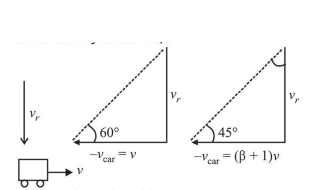# When a car si at rest, its driver sees raindrops falling on it vertically.

Question:

When a car si at rest, its driver sees raindrops falling on it vertically. When driving the car with speed $v$, he sees that raindrops are coming at an angle $60^{\circ}$ from the horizontal. On furter increasing the speed of the car to $(1+\beta) v$, this angle changes to $45^{\circ}$. The value of $\beta$ is close to:

1. (1) $0.50$

2. (2) $0.41$

3. (3) $0.37$

4. (4) $0.73$

Correct Option: , 4

Solution:

(4) The given situation is shown in the diagram. Here $v_{r}$ be the velocity of rain drop.When car is moving with speed $v$,

$\tan 60^{\circ}=\frac{v_{r}}{v}$ ...(i)

When car is moving with speed $(1+\beta) v$,

$\tan 45^{\circ}=\frac{v_{r}}{(\beta+1) v}$ ....(ii)

Dividing (i) by (ii) we get,

$\sqrt{3} v=(\beta+1) v \Rightarrow \beta=\sqrt{3}-1=0.732$